# Resources tagged with: Angle properties of polygons

Filter by: Content type:
Age range:
Challenge level:

### There are 31 results

Broad Topics > Angles, Polygons, and Geometrical Proof > Angle properties of polygons### Tessellating Hexagons

##### Age 11 to 14Challenge Level

Which hexagons tessellate?### Convex Polygons

##### Age 11 to 14Challenge Level

Show that among the interior angles of a convex polygon there cannot be more than three acute angles.### No Right Angle Here

##### Age 14 to 16Challenge Level

Prove that the internal angle bisectors of a triangle will never be perpendicular to each other.##### Age 11 to 16Challenge Level

Draw some quadrilaterals on a 9-point circle and work out the angles. Is there a theorem?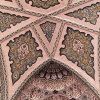### Angles Inside

##### Age 11 to 14Challenge Level

Draw some angles inside a rectangle. What do you notice? Can you prove it?### Polygon Rings

##### Age 11 to 14Challenge Level

Join pentagons together edge to edge. Will they form a ring?### Getting an Angle

##### Age 11 to 14Challenge Level

How can you make an angle of 60 degrees by folding a sheet of paper twice?### Polygon Pictures

##### Age 11 to 14Challenge Level

Can you work out how these polygon pictures were drawn, and use that to figure out their angles?##### Age 14 to 16Challenge Level

Make five different quadrilaterals on a nine-point pegboard, without using the centre peg. Work out the angles in each quadrilateral you make. Now, what other relationships you can see?### Semi-regular Tessellations

##### Age 11 to 16Challenge Level

Semi-regular tessellations combine two or more different regular polygons to fill the plane. Can you find all the semi-regular tessellations?### Bow Tie

##### Age 11 to 14Challenge Level

Show how this pentagonal tile can be used to tile the plane and describe the transformations which map this pentagon to its images in the tiling.### Making Sixty

##### Age 14 to 16Challenge Level

Why does this fold create an angle of sixty degrees?### A Sameness Surely

##### Age 14 to 16Challenge Level

Triangle ABC has a right angle at C. ACRS and CBPQ are squares. ST and PU are perpendicular to AB produced. Show that ST + PU = AB### Terminology

##### Age 14 to 16Challenge Level

Given an equilateral triangle inside an isosceles triangle, can you find a relationship between the angles?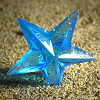### Star Polygons

##### Age 11 to 14Challenge Level

Draw some stars and measure the angles at their points. Can you find and prove a result about their sum?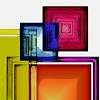### Angles in Three Squares

##### Age 14 to 16Challenge Level

Drawing the right diagram can help you to prove a result about the angles in a line of squares.### Which Solids Can We Make?

##### Age 11 to 14Challenge Level

Interior angles can help us to work out which polygons will tessellate. Can we use similar ideas to predict which polygons combine to create semi-regular solids?### Pent

##### Age 14 to 18Challenge Level

The diagram shows a regular pentagon with sides of unit length. Find all the angles in the diagram. Prove that the quadrilateral shown in red is a rhombus.### Tricircle

##### Age 14 to 16Challenge Level

The centre of the larger circle is at the midpoint of one side of an equilateral triangle and the circle touches the other two sides of the triangle. A smaller circle touches the larger circle and. . . .### Arclets Explained

##### Age 11 to 16

This article gives an wonderful insight into students working on the Arclets problem that first appeared in the Sept 2002 edition of the NRICH website.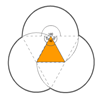### Triangles and Petals

##### Age 14 to 16Challenge Level

An equilateral triangle rotates around regular polygons and produces an outline like a flower. What are the perimeters of the different flowers?### Floored

##### Age 14 to 16Challenge Level

A floor is covered by a tessellation of equilateral triangles, each having three equal arcs inside it. What proportion of the area of the tessellation is shaded?### Darts and Kites

##### Age 14 to 16Challenge Level

Explore the geometry of these dart and kite shapes!### Gibraltar Geometry

##### Age 11 to 14Challenge Level

Take a look at the photos of tiles at a school in Gibraltar. What questions can you ask about them?### At a Glance

##### Age 14 to 16Challenge Level

The area of a regular pentagon looks about twice as a big as the pentangle star drawn within it. Is it?### Pentakite

##### Age 14 to 18Challenge Level

ABCDE is a regular pentagon of side length one unit. BC produced meets ED produced at F. Show that triangle CDF is congruent to triangle EDB. Find the length of BE.### Dodecawhat

##### Age 14 to 16Challenge Level

Follow instructions to fold sheets of A4 paper into pentagons and assemble them to form a dodecahedron. Calculate the error in the angle of the not perfectly regular pentagons you make.### LOGO Challenge 4 - Squares to Procedures

##### Age 11 to 16Challenge Level

This LOGO Challenge emphasises the idea of breaking down a problem into smaller manageable parts. Working on squares and angles.### Logo Challenge 3 - Star Square

##### Age 7 to 16Challenge Level

Creating designs with squares - using the REPEAT command in LOGO. This requires some careful thought on angles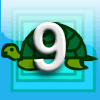### First Forward Into Logo 9: Stars

##### Age 11 to 18Challenge Level

Turn through bigger angles and draw stars with Logo.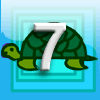### First Forward Into Logo 7: Angles of Polygons

##### Age 11 to 18Challenge Level

More Logo for beginners. Learn to calculate exterior angles and draw regular polygons using procedures and variables.